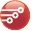# Problem creating grid in code behind

GridTop achievementsRank 2
Web Services asked on 16 May 2012, 11:06 PM
I'm having trouble with post backs on a grid where the columns are created in the code behind. Essentially the columns that go in this grid come from a table, but an administrator has another page where they select what columns from the table show in the grid. So, I have table that stores column names and I dynamically add the columns with their header text and data field id based on this table that stores the columns that need to show. I then dynamically create the select statement, set that as the select command to a sqldatasource and bind it. When you load the page initially everything works fine. However, when you click some paging or sorting, it adds a bunch of extra columns. You can see the before and after here
http://imgur.com/a/gO28G#nOVo1

Here is my ascx
`<``telerik:radgrid` `ID``=``"gradeGrid"` `runat``=``"server"` `AllowPaging``=``"True"` `GridLines``=``"None"`
`            ``CellPadding``=``"5"` `AutoGenerateColumns``=``"false"` `Width``=``"850px"` `Skin``=``"Metro"` `>`
`        ``</``telerik:radgrid``>`
`        ``<``asp:SqlDataSource` `ID``=``"SqlDataSource1"` `runat``=``"server"` `ConnectionString="<%\$ ConnectionStrings:connection %>">`
`        ``</``asp:SqlDataSource``>`

here is my code behind
`Dim` `conn = System.Configuration.ConfigurationManager.ConnectionStrings(``"connection"``).ToString`

`   ``'this sub adds a column to the rad grid`
`   ``Protected` `Friend` `Sub` `addColumn(``ByVal` `dataField ``As` `String``, ``ByVal` `header ``As` `String``)`

`       ``Dim` `col ``As` `New` `GridBoundColumn`
`       ``col.DataField = dataField`
`       ``col.HeaderText = header`
`       ``gradeGrid.MasterTableView.Columns.Add(col)`

`   ``End` `Sub` `'addColumn`

`   ``Protected` `Sub` `Page_Load(``ByVal` `sender ``As` `Object``, ``ByVal` `e ``As` `System.EventArgs) ``Handles` `Me``.Load`

`       ``If` `(``Not` `Page.IsPostBack) ``Then`

`           ``bindGrid()`
`           ``gradeGrid.DataBind()`

`       ``End` `If` `'if its not a  post back`

`   ``End` `Sub`

`   ``'this sub gets the data for the rad grid`
`   ``Protected` `Sub` `bindGrid()`

`       ``Dim` `reader ``As` `SqlDataReader`
`       ``'add the columns we know about`
`       ``addColumn(``"lgrade"``, ``"Letter Grade"``)`
`       ``addColumn(``"ngrade"``, ``"Percent Grade"``)`
`       ``addColumn(``"Course Code"``, ``"sectno"``)`
`       ``addColumn(``"Completed"``, ``"enddate"``)`

`       ``Dim` `itemSql = ``"SELECT lgrade, ngrade, sectno, enddate, "`
`       ``'now find all the other ones we need`
`       ``Dim` `sql = ``"SELECT columnName, label FROM hstuclsDefinedColumns ORDER BY position"`
`       ``Using myconn ``As` `New` `SqlConnection(conn)`

`           ``myconn.Open()`

`           ``reader = ``New` `SqlCommand(sql, myconn).ExecuteReader()`
`           ``If` `(reader.HasRows) ``Then`

`               ``While` `reader.Read()`
`                   ``addColumn(reader(``"columnName"``), reader(``"label"``))`
`                   ``itemSql &= ``"["` `& reader(``"columnName"``) & ``"]"` `& ``", "`
`               ``End` `While` `'reader.read`
`               ``reader.Close()`
`           ``End` `If` `'if reader.hasrows`

`           ``itemSql &= ``" 'blah' FROM hstucls WHERE stuid = "` `& MyFunctions.getStudentId`

`           ``myconn.Close()`
`       ``End` `Using ``'using myconn`

`       ``SqlDataSource1.SelectCommand = itemSql`

`       ``gradeGrid.DataSource = SqlDataSource1`

`   ``End` `Sub` `'bindGrid`

`   ``Protected` `Sub` `gradeGrid_NeedDataSource(``ByVal` `sender ``As` `Object``, ``ByVal` `e ``As` `Telerik.Web.UI.GridNeedDataSourceEventArgs) ``Handles` `gradeGrid.NeedDataSource`
`       ``bindGrid()`
`   ``End` `Sub`

I'm sure it's something stupid, I just don't see what it is. Thanks!

## 1 Answer, 1 is accepted

0Web Services
Top achievementsRank 2
answered on 17 May 2012, 06:26 PM
Found my problem via another forum. Had to change

`Dim` `col ``As` `New` `GridBoundColumn`
`col.DataField = dataField`
`col.HeaderText = header`
`gradeGrid.MasterTableView.Columns.Add(col)`

to
`Dim` `col ``As` `New` `GridBoundColumn`
`gradeGrid.MasterTableView.Columns.Add(col)`
`col.DataField = dataField`
`col.HeaderText = header`

Move the columns.add method per your example. Why does it cause issues on the post back but not on the page load?

Tags
GridRank 2Rank 2